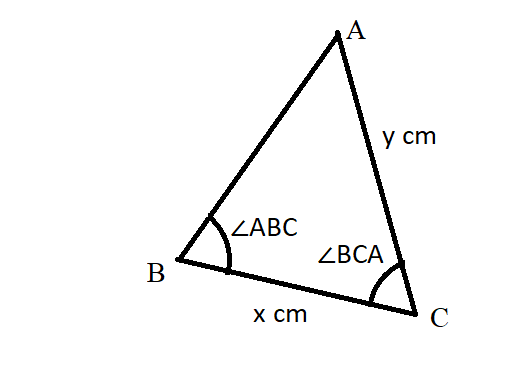# If the measure of an angle is 23°17′18′′, what is the measure of its supplement?Question
Non-right triangles and trigonometryIf the measure of an angle is 23°17′18′′, what is the measure of its supplement?2021-03-07
Two supplementary angles have angle measures having a sum of 180°. So, the other angle can be found by subtracting the given angle from 180°:
180°−23°17′18′′=179°59′60′′°−23°17′18′′=156°42′42′′

### Relevant QuestionsThe measure of the complement of an angle is three times the measure of the angle. What is the measure of each angle?In $$\displaystyle△{P}{Q}{R},{m}∠{P}={46}{x}$$ and $$\displaystyle{m}∠{R}={7}{x}$$,
a. Write and solve an equation to find the measure of each angle.
b. Classify the triangle based on its angles.[Triangle] Determine the measure of each interior angle of △MAN and classify the triangle. m m /trnglMAN is a(n)_______________ triangle.In triangle ABC, angle B is five times as large as angle A. The measure of angle C is 2° less than that of angle A. Find the measures of the angles. (Hint: The sum of the angle measures is 180°.)A man was parasailing above a lake at an angle of elevation of 32° from a boat, as modeled in the diagram, If 129.5 meters of cable connected the boat to the parasail, approximately how many meters above the lake was the man?
(1) 68.6
(2) 80.9
(3) 109.8
(4) 244.4Suppose that $$\displaystyle{m}\angle{B}{C}{A}={70}^{\circ}$$, and x=33 cm and y = 47 cm. What is the degree measure of $$\displaystyle\angle{A}{B}{C}$$?Given a triangles. One angle is $$\displaystyle{42}^{\circ}$$. Find the other angles if they're equal.if $$\displaystyle\angle{c}=\angle{b}{\quad\text{and}\quad}\angle{a}={4}\angle{b}$$, find the value of each angle.PQRS is a parallelogram. $$\displaystyle{m}∠{Q}={11}{x}+{13}$$ and $$\displaystyle{m}∠{S}={15}{x}-{23}$$. Find the measure of $$\displaystyle∠{P}$$.